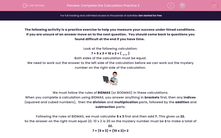# Complete the Calculation Practice 2

In this practice exercise, students will complete a calculation by adding in the missing number so that both sides are equal using BIDMAS, under timed conditions.This content is premium and exclusive to EdPlace subscribers.Key stage:  KS 2

Curriculum topic:   Exam-Style Questions: Verbal Reasoning

Curriculum subtopic:   Exam-Style Questions: Complete the Calculation

Difficulty level:#### Worksheet Overview

If you are unsure of an answer move on to the next question.  You should come back to questions you found difficult at the end if you have time.

Look at the following calculation:

7 + 5 x 3 = 10 x 2 + ( __ )

Both sides of the calculation must be equal.

We need to work out the answer to the left side of the calculation before we can work out the mystery number on the right side of the calculation.We must follow the rules of BIDMAS (or BODMAS) in these calculations.

When you complete a calculation using BIDMAS, you answer anything in brackets first, then any indices (squared and cubed numbers),  then the division and multiplication parts, followed by the addition and subtraction parts.

Following the rules of BIDMAS, we must calculate 5 x 3 first and then add 7. This gives us 22.

So the answer on the right must equal 22. 10 x 2 is 20 so the mystery number must be 2 to make a total of 22.

7 + (5 x 3) = (10 x 2)+ 2

Now it's time to begin this practice exercise.  Good luck!

### What is EdPlace?

We're your National Curriculum aligned online education content provider helping each child succeed in English, maths and science from year 1 to GCSE. With an EdPlace account you’ll be able to track and measure progress, helping each child achieve their best. We build confidence and attainment by personalising each child’s learning at a level that suits them.

Get started